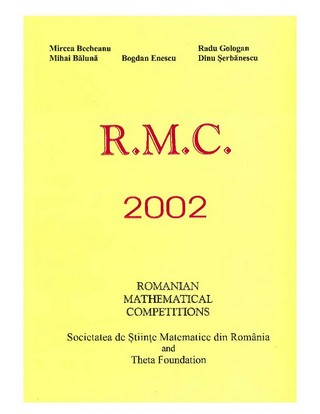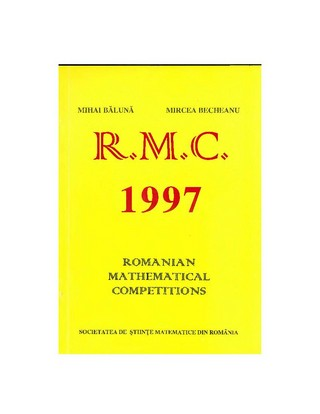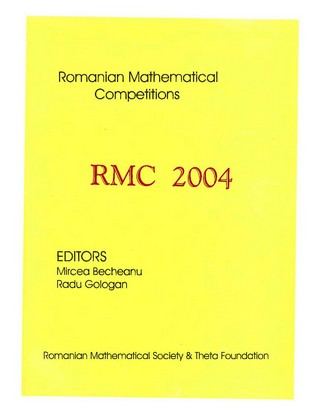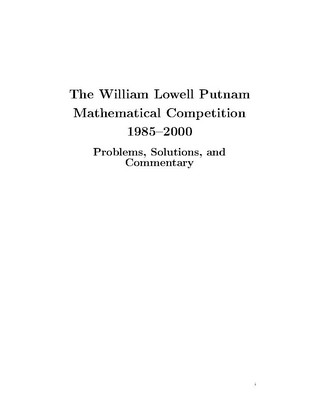Book Toankho

Sách hay - Sách đẹp

Browsing:

Tag: Competition

Romanian Mathematical Competitions 2007Romanian Mathematical Competitions 1998Romanian Mathematical Competitions 2002Combinatorial Problems in Mathematical Competitions (Mathematical Olympiad Series Vol. 4)This book focuses on combinatorial problems in mathematical competitions. It provides basic knowledge on how to solve combinatorial problems in mathematical competitions, and also introduces important solutions to combinatorial problems and some typical problems with often-used solutions. Some enlightening and Read more…

Romanian Mathematical Competitions 1997New World Selection 1 of Mathematical CompetitionsNew World Selection of Mathematical Competitions by Saing Darareaksmey Download ToanKho:http://lk.libvui.com/wMuwQ http://megaurl.io/Gj1g

Romanian Mathematical Competitions 2003Romanian Mathematical Competitions 2004Romanian Mathematical Competitions 1996